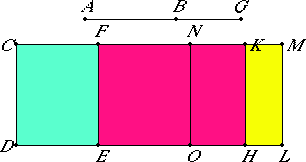# Proposition 101

The square on the straight line which produces with a rational area a medial whole, if applied to a rational straight line, produces as breadth a fifth apotome.

Let AB be the straight line which produces with a rational area a medial whole, and CD a rational straight line, and to CD let CE be applied equal to the square on AB and producing CF as breadth.

I say that CF is a fifth apotome.

X.77

Let BG be the annex to AB. Then AG and GB are straight lines incommensurable in square which make the sum of the squares on them medial but twice the rectangle contained by them rational.To CD apply CH equal to the square on AG, and KL equal to the square on GB. Then the whole CL equals the sum of the squares on AG and GB.

But the sum of the squares on AG and GB together is medial, therefore CL is medial.

X.22

And it is applied to the rational straight line CD producing CM as breadth, therefore CM is rational and incommensurable with CD.

II.7

Since the whole CL equals the sum of the squares on AG and GB, and, in these, CE equals the square on AB, therefore the remainder FL equals twice the rectangle AG by GB.

Bisect FM at N, and draw NO through N parallel to either of the straight lines CD or ML. Then each of the rectangles FO and NL equals the rectangle AG by GB.

And, since twice the rectangle AG by GB is rational and equal to FL, therefore FL is rational.

X.20

And it is applied to the rational straight line EF producing FM as breadth, therefore FM is rational and commensurable in length with CD.

Now, since CL is medial, and FL rational, therefore CL is incommensurable with FL.

But CL is to FL as CM is to MF, therefore CM is incommensurable in length with MF.

X.73

And both are rational, therefore CM and MF are rational straight lines commensurable in square only. Therefore CF is an apotome.

I say next that it is also a fifth apotome.

We can prove similarly that the rectangle CK by KM equals the square on NM, that is, the fourth part of the square on FM.

And, since the square on AG is incommensurable with the square on GB, while the square on AG equals CH, and the square on GB equals KL, therefore CH is incommensurable with KL.

But CH is to KL as CK is to KM, therefore CK is incommensurable in length with KM.

X.18

Since CM and MF are two unequal straight lines, and a parallelogram equal to the fourth part of the square on FM and deficient by a square figure has been applied to CM, and divides it into incommensurable parts, therefore the square on CM is greater than the square on MF by the square on a straight line incommensurable with CM.

X.Def.III.5

And the annex FM is commensurable with the rational straight line CD set out, therefore CF is a fifth apotome.

Therefore, the square on the straight line which produces with a rational area a medial whole, if applied to a rational straight line, produces as breadth a fifth apotome.

Q.E.D.

## Guide

This proposition is used in X.111.